# NCERT Solutions class-11 Maths Exercise 5.1## myCBSEguide App

CBSE, NCERT, JEE Main, NEET-UG, NDA, Exam Papers, Question Bank, NCERT Solutions, Exemplars, Revision Notes, Free Videos, MCQ Tests & more.

Exercise 5.1

Express each of the complex numbers given in the exercises 1 to 10 in the form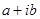:

1.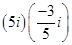Ans. Here=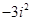=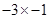=3

2.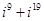Ans. Here=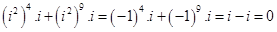3.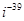Ans.=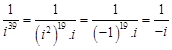=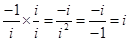4.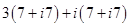Ans. Here=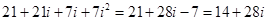5.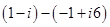Ans. Here=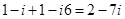6.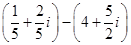Ans. Here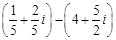=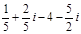=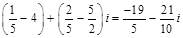7.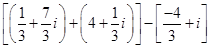Ans. Here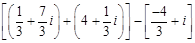=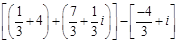=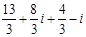=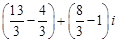=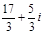8.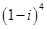Ans. Here=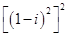=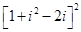=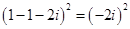=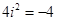9.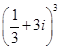Ans. Here=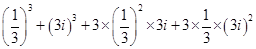=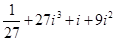=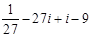=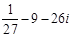=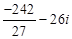10.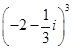Ans. Here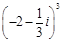=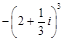=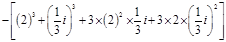=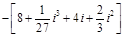=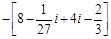=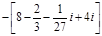=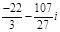Find the multiplicative inverse of each of the complex numbers given in the exercises 11 to 13.

11.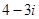Ans. Multiplicative Inverse of=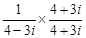=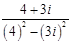=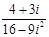=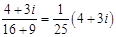12.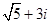Ans. Multiplicative Inverse of=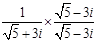=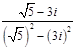=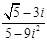=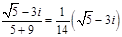13.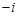Ans. Multiplicative Inverse of=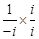=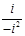=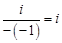14. Express the following expression in the form of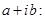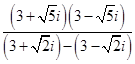Ans. Here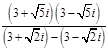=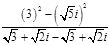=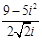=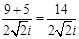=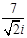=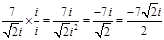1. Very good